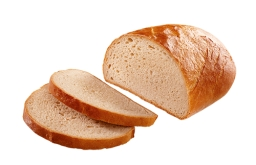# Kilogram 7162

Mom paid CZK 132.50 for the purchase. She bought bread, 5 kg apples, and three liters of milk. We know that bread costs twice as much as milk, and a kilogram of apples costs 6.50 CZK less than milk. How many CZKs did milk and bread and a kilogram of apples cost?

a =  30.4 Sk
b =  15.2 Sk
c =  8.7 Sk

### Step-by-step explanation:

a + 5·a/2+3·(a/2-6.50) = 132.50

10a = 304

a = 304/10 = 30.4

a = 152/5 = 30.4

Our simple equation calculator calculates it.Did you find an error or inaccuracy? Feel free to write us. Thank you!

Tips for related online calculators
Need help calculating sum, simplifying, or multiplying fractions? Try our fraction calculator.
Do you have a linear equation or system of equations and looking for its solution? Or do you have a quadratic equation?